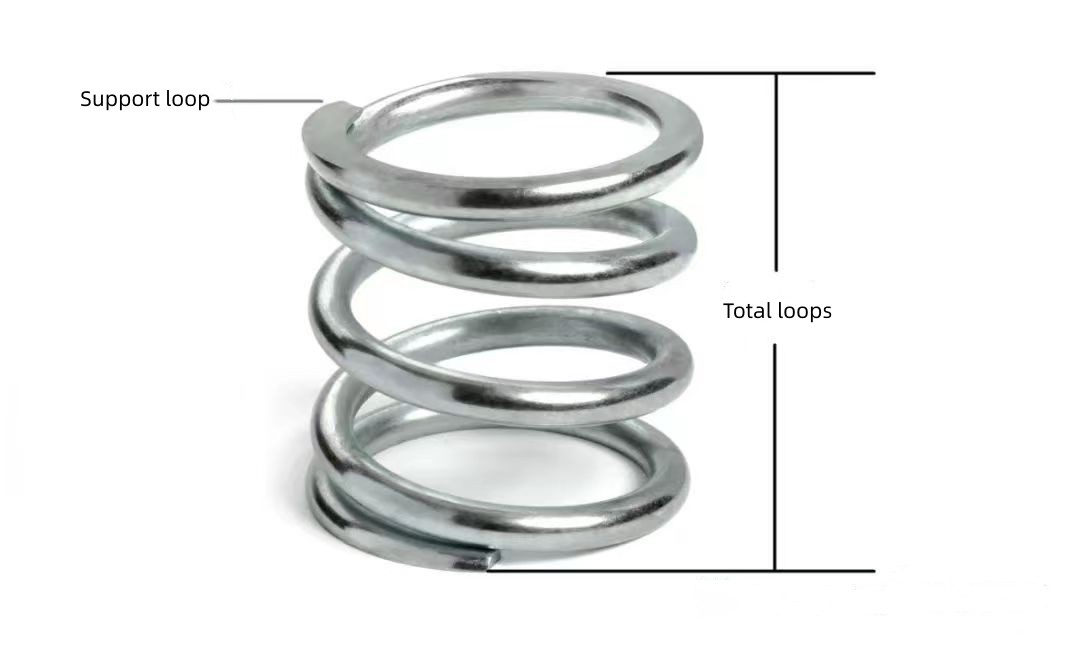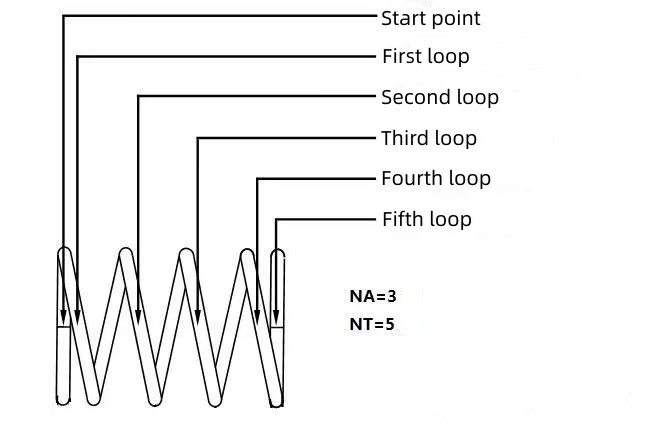What is the effective number of turns for a Nitinol spring?

The number of turns from one end of the compression spring to the other end is called the total number of turns Nt.

In the total number of turns, the number of turns that can maintain the same pitch at the same time as the helical part that acts as a spring is called the effective number of turns Na.

The helical part that does not act as a spring is called the support ring Nia.

The effective number of turns of the spring Na = the total number of turns Nt – the support ring Nia

It is calculated according to the structure.For regular compression springs

Effective number of turns Na = total number of turns Nt – number of support turns Nia

For conventional extension springs and torsion springsEffective number of turns Na = total number of turns Nt (all turns are involved in the spring action of tension or torsion)

General speaking, the algorithm for the effective number of coils of the compression spring is the total number of coils minus the number of coils that will not deform due to contact up and down, and generally subtract 1 coil above and below. The effective number of turns for torsion and extension springs is the total number of turns.

However, normally it is difficult to have a clear boundary between the support circle and the effective number of circles, and it can only be checked visually. In order to make the spring force value meet the requirements of the drawing, the manufacturer often adjusts the effective number of turns. The number of valid laps is generally controlled as a reference item.